[BACK]Computer Modeling in Engineering & SciencesDOI: 10.32604/cmes.2022.020066

ARTICLE

On Soft Pre-Rough Approximation Space with Applications in Decision Making

1Department of Mathematics, College of Science and Arts, Najran University, Najran, 66445, Saudi Arabia
2Department of Mathematics and Statistics, College of Science, Taif University, Taif, 21944, Saudi Arabia
*Corresponding Author: M. El Sayed. Email: mebadria@nu.edu.sa
Received: 02 November 2021; Accepted: 20 January 2022

Abstract: A soft, rough set model is a distinctive mathematical model that can be used to relate a variety of real-life data. In the present work, we introduce new concepts of rough set based on soft pre-lower and soft pre-upper approximation space. These concepts are soft pre-rough equality, soft pre-rough inclusion, soft pre-rough belonging, soft pre-definability, soft pre-internal lower, and soft pre-external lower. We study the properties of these concepts. Finally, we use the soft pre-rough approximation to illustrate the importance of our method in decision-making for Chikungunya medical illnesses. In reality, the impact factors of Chikungunya's medical infection were determined. Moreover, we develop two new algorithms to address Chikungunya virus issues. Our proposed approach is sensible and effective.

Keywords: Soft rough set; soft pre-rough set approach; soft pre-internal lower and soft pre-external upper; soft nowhere dense set and Chikungunya medical application; intelligence discovery

1  Introduction

The chikungunya virus is transmitted to humans by the bite of an infected mosquito. Fever and joint discomfort are the most typical symptoms of infection. Headache, muscle soreness, joint swelling, and rash are some of the other symptoms. The chikungunya virus was first discovered in the Americas in late 2013 on the Caribbean islands. The number of research articles published has exploded at a quick pace, particularly in mathematics. Several proposals were given for solving real-world problems with mathematical methodologies and relevant formulas to assist decision-makers in making the best decisions possible. To deal with challenges that are uncertain ().

To reduce the uncertainty and vagueness of knowledge, Molodtsov  developed soft sets; as a novel technique for modeling uncertainty, creating the fundamentals of the corresponding theory. He has demonstrated how this theory may be used to solve a variety of practical issues in economics, engineering, social science, and medicine, among other fields. Many researchers introduced several applications of soft set theory (). We are implementing new classes of concepts in this paper based on soft pre-rough set .

In our everyday lives, we are often constantly faced with challenges that necessarily require rational decision-making. Yet, we get uncertain about the correct answer in several of these situations. We must consider different criteria related to the solution in order to arrive at the best possible solution to these problems. For this, in our paper we can use the best mathematical tool namely soft, rough set theory in decision making. The classical soft sets were also applied to fuzzy soft sets by the same authors . Maji et al. [15,16] discussed the application of soft set theory to a problem of decision-making, and the implementation of soft set theory was explored. They created soft, rough approximations, soft, rough sets, and several related concepts based on this granulation structure. The soft rough sets model established by Feng et al.  is generalized in this paper. The goal was to exert some influence on the ongoing issue. This approximation is a generalization to Feng et al. ; we have demonstrated that our approach is more accurate and comprehensive than that of Feng et al.  defined the soft rough model as a generalization of the Pawlak rough models (SRs) . Since then, many researchers have further studied RS as in the following published articles . The authors in  introduced a new approach coupled with applications based on relation in soft generalized topological spaces and they studied their properties. Mathematical modeling of vagueness and ambiguity is becoming an increasingly important in a variety of fields of study. Another statistical tool that has been used in many aspects of life is regression analysis [25,26].

In this paper, we used this approximation to define many new concepts based on it, namely soft pre-rough belonging, soft pre-rough inclusion, soft pre-rough definability, soft pre-rough equality, and we studied the properties of these concepts. The present approximations are significant not just because they reduce or eliminate border areas. Finally, we will introduce an application in decision making of these concepts. At the end of the paper, we will present an algorithm that can be used to decide on an information system to show the importance of this approximation.

Here's how the document goes: Originality starts from Sections 2 and 3, and the preliminary basic concepts are covered. Sections 4 and 5 discuss both the rough and soft sets, as well as the use of soft pre-rough for each subclass of characteristics in information systems and applications. In Section 6, a few concluding notes provide a discussion and recommendations for future scope.

2  Preliminaries

We offer some fundamental concepts and outcomes that are utilized in the paper:

Definition 2.1  Let S=(Γ,A)be a soft set over upon Uand A=(U,S)be a space. Consequently, the soft “pre-lower” and “pre-upper” approximations of any subset XU are defined respectively, by: the soft pre-lower Bs(X)=XN_(N¯(X)) and the soft pre-upper Bs(X)=XN¯(N_(X)).

We refer to (Bs(X),Bs(X)) as “soft pre-rough approximations” with respect to As.

Definition 2.2  Assuming that As=(U,S)be a space and XU. Consequently, the soft “pre- positive, pre-negative, pre-boundary” regions and the “pre-accuracy” of the soft pre-approximations are defined respectively by: POS(X)=BS(X), NEG(X)=UBS(X), BND(X)=BS(X)BS(X) and μ=|BS(X)BS(X)|, where BS(X)0.

Clearly, if BS(X)=BS(X),BND(X)=φ and μ=1. Then XU it is called “soft pre-definable” or “soft pre-exact” set; otherwise X it is called a “soft pre-rough” set.

The principal purpose of the following outcomes is to present and superimpose the fundamental features of soft pre-rough approximations BS(X) and BS(X).

Proposition 2.1  Let S=(Γ,A) be a soft set upon U and As=(U,S) be a space. Thus, the soft pre-lower and pre-upper approximations of XU satisfy the following properties:

(i) Bs(φ)=Bs(φ)=φ

(ii) Bs(U)=eAf(e),Bs(U)=U

(iii) IXY then Bs(X)Bs(Y)

(iv) If XY, then Bs(X)Bs(Y)

(v) Bs(XY)=Bs(X)Bs(Y)

(vi) Bs(XY)Bs(X)Bs(Y)

(vii) Bs(XY)Bs(X)Bs(Y)

(viii) Bs(XY)=Bs(X)Bs(Y)

3  Approaches on Soft Set

3.1 Soft Pre-Definability of Sets

In this section, we presented the definitions of definability of sets by using soft pre-rough approximation, namely, soft pre-internal upper, soft pre-external lower of a set A, and we denote to the soft pre-internal upper, soft pre-external lower of a set A by InteS(A) and ExteS(A), respectively.

The definition that follows introduces new concepts of definability for a subset AX in soft pre-rough approximation space.

Definition 3.1 Assuming S=(Γ,A) be a soft set upon U and As=(U,S) be a space. Then, the soft pre-lower and pre-upper approximations of and AX,Aφ. Then A is called.

i.   Soft pre-internally definable if and only if ExteS(A)=φ and InteS(A)φA=appS(A).

ii.  Soft pre-externally definable if and only if InteS(A)=φ and ExteS(A)φBS(A)=A.

iii. 6Soft pre-roughly undefinable if and only if BS(A)BS(A)A. This means that some elements of X belong to A and some elements belongs to AC.

iv.  Soft pre-exact (briefly Soft pre-exact) set if and only if A=BS(A)=BS(A) and hence bS(A)=φ.

Definition 3.2 Let S=(Γ,A) be a soft set upon Uand As=(U,S) a soft approximation space. and AU,Aφ. Consequently A is called:

i.   Soft pre-internally definable (resp. Soft pre-externally definable and soft pre-exact).

ii.  Soft pre-internally definable set if and only if A=BS(A), i.e., ExteS(A)=φ.

iii. Soft pre-externally definable set if and only if A=appS(A), i.e., InteS(A)=φ.

Definition 3.3 Let S=(Γ,A)be a soft set upon, As=(U,S) be a soft space. Then, the soft pre-lower and pre-upper approximations of and AU,Aφ. Then A is called:

i.   Soft pre-external lower (briefly Exstep(A)) if Exstep(A)=ABS(A).

ii.  Soft pre-external lower (briefly Ixstep(A) ) if Ixstep(A)=BS(A)A.

iii. Soft pre-exterior (briefly extp(A) ) if extp(A)=UBS(A).

Proposition 3.1 Let S=(Γ,A)be a soft set upon U, As=(U,S) be a soft space, A,BU,A,Bφ. Then, the next announcements are held:

i.   If AB, then extp(B)extp(A).

ii.  extp(AB)=extp(A)extp(B).

iii. extp(AB)extp(A)extp(B).

Proof.

i.   Since BS(A)BS(B) by taken the complement for both sides, we get XBS(B)XBS(A) and hence extp(B)extp(A).

ii.  Since extp(AB)=UBS(AB)=(UBS(A))(UBS(B))=extp(A)extp(B) then extp(AB)=extp(A)extp(B).

iii. Since extp(AB)=UBS(AB)(UBS(A))(UBS(B)=extp(A)extp(B). Then extp(AB)extp(A)extp(B).

The following example shows the equality in (iii) of the above proposition.

Example 3.1 Suppose that S=(Γ,A) be a soft set upon U, As=(U,S) a soft approximation space, where, U={χ1,χ2,χ3,χ4,χ5}, E={δ1,δ2,δ3,,δ6} and A={δ1,δ2,δ3,δ4}E, so that (Γ,A)={(δ1,{χ1}),(δ2,{χ2,χ5}),(δ3,{χ3}),(δ4,{χ5})}. Now let X={χ1,χ2,χ3},Y={χ3,χ4,χ5}. Then, we get Bs(X)={χ1,χ2,χ3} and Bs(Y)={χ2,χ3,χ4,χ5} which implies extpX={χ4,χ5} and ext(Y)={χ1}. Hence, ext(X)ext(Y)={χ1,χ4,χ5}(i)since apprs(XY)={χ3}. Then ext(XY)={χ1,χ2,χ4,χ5}(ii) thus from (i), (ii) we get extp(AB)extp(A)extp(B).

Proposition 3.2 Let be full soft set upon U, As=(U,S) be a soft space, XU,Xφ. Then,

i.  (BS(X))CBS(XC)

ii. NEG(X)=(BS(X))CBS(X))C

Proof.

i.  Since BS(X)=XBS(BS(X)) then BS(X))c=(XN(N(X)))c=XC(N(N(X)))cXCN((N(X))c)XCN(N(X)C)=BS(X)C Then (BS(X))CBS(XC).

ii. Since NEG(X)=(BS(X))Cand since BS(X)=XN(N(X) thus (BS(X))C=(XN(N(X)))C=XC(N(N(X))CXC(N(N(X))CXCN(N(XC)=BS(XC).

Proposition 3.3 Assumption that S=(Γ,A) be a soft set upon, As=(U,S) be a soft space, AX,Aφ. Then:

i.   A is soft pre-exact set then A is soft pre-internally and soft pre-externally definable.

ii.   A is soft pre-roughly un-definable set if and only if A is neither soft pre-internally nor soft pre-externally definable.

Proof.

i.   Let A be a soft pre-exact. Then, A=BS(A)=BS(A). Since A=BS(A). Then ExtS(A)=φ. And hence A is soft pre-internally definable since A=BS(A). Then IntS(A)=φ therefore A is soft pre-externally definable.

ii.   Let A be a soft pre-rough undefinable set hence BS(A)BS(A)A. Then A is neither soft pre-internally and soft pre-externally definable. Conversely, it is obvious.

Proposition 3.4 Assumption that S=(Γ,A) be a soft set over upon U, As=(U,S) be a space, AX,Aφ. If A is soft pre-roughly undefinable the BS(A)BS(A)A.

Proof. Let A be a soft pre-roughly undefinable. Therefore A is not soft pre-exact and hence BS(A)BS(A)A.

Proposition 3.5 Assumption that S=(Γ,A) be a soft set over upon U, As=(U,S) be a space, AX,Aφ. Thence A is soft pre-roughly undefinable if and only if BS(A)BS(A)A.

Proof. Let A be a soft pre-roughly undefinable. Thus, is not soft pre-exact then BS(A)BS(A)A. Conversely, let BS(A)BS(A)A. Thus, A is not soft pre-exact. Therefore, A is not simply open set and BS(A)BS(A)A. Hence, A is soft pre-roughly undefinable.

3.2 Soft Pre-Rough Belonging

In this section, we introduce new definitions on rough membership relation which indicates belonging to the elements of the set by using soft pre lower and soft pre upper approximations and we are studying some of their properties.

Definition 3.4 Assumption that S=(Γ,A) be a soft set over upon U, As=(U,S) be a space and upper belong as follows:

i.   a is soft pre-lower belonging to A (briefly apA ) iff aBS(A).

ii.   a is soft pre-upper belonging to A (briefly apA ) iff aBS(A).

Remark 3.1 Assumption that S=(Γ,A) be a soft set over upon U and As=(U,S) be a space. and AU,Aφ. Then, for each aU we have:

i.   If apA, then apA.

ii.   If aRA, then apA.

iii.   If apA, then aRA.

The next example clarifies the above remark. Also, this example shows the concepts of soft pre-lower belong and soft pre lower belong which we shall use in the following application.

Example 3.2 Assumption that S=(Γ,A) be a soft set upon U, As=(U,S) is a space, where U={χ1,χ2,χ3,χ4,χ5,χ6},E={δ1,δ2,.,δ6} and A={e1,e2,e3,e4}E such that (Γ,A)={(δ1,{χ1,χ6}),(δ2,{χ3}),(δ3,φ),(δ4,{χ1,χ2,χ5})}. Now let X={χ3,χ4,χ5} but Bs(X)={χ3,χ5} and Bs(X)={χ3,χ4,χ5}. It is clear that an element χ4X, but χ4pX hence χ4Bs(X). Also, the same element χ4Bs(X) and hence χ4pX.

Proposition 3.6 Assumption that S=(Γ,A) be a soft set upon U, As=(U,S) is a space, and N,H^U. Consequently the next assumptions are valid:

i.   If NH^, then (zPNzPH^ and zPNzPH^).

ii.   If zP(NH) then zPN and zPH^.

iii.   zP(NH^)zPN or zPH^.

iv.   If zPN or zPH^ Then zP(NH^).

v.   zP(NH^)zPN and zPH^.

vi.   zPNCzPN.

vii.   zPNCzPN.

Proof.

i.   Let NH^. Thus BS(N)BS(H^) and BS(N)BS(H^) if zpN. Then zBS(N) but BS(N)BS(H^). Thus zBS(H^) and hence zpH^. Similarly we can prove the second part.

ii.   Let zP(NH^). Thus zBS(NH^) and hence z(BS(N)BS(H^)). This tends to zBS(N) and zBS(H^). Then zPA and zPH^.

iii.   Let zP(NH^). Thus zBS(NH^)zBS(N) or zBS(H^)zPN or zPH^.

iv.   Let zpN or zpH^. Then zBS(N) or zBS(H^). Hence zBS(N)BS(H^)BS(NH^) thus zBS(NH^). Therefore zp(NH^).

v.   Let zp(NH^). Thus zBS(NH^) but BS(NH^)=BS(N)BS(H^) . Then zBS(N) and zBS(H^)zpN and zpH^.

3.3 Soft Pre-Rough Equality

We will introduce in this section a new class of equality by using soft pre-lower and soft pre -upper approximations namely, soft pre lower equal, soft pre upper equal and approximations of any two sets and we study some of their properties.

Definition 3.5 Assuming that S=(Γ,A) be a soft set upon U, AS=(U,S) is a space and A,H^U, then:

i.   The sets A and H^ are soft pre lower equal (briefly ApH^ ) if BS(A)=BS(H^)

ii.   The sets A and H^ are soft upper equal (briefly ApH^) if BS(A)=BS(H^).

iii.   The sets A and H^ are soft approximations (briefly ApH^) if ApH^ and ApH^.

Proposition 3.7 Assumption that S=(Γ,A) be a soft set upon U,As=(U,S) is a space, Consequently and W^,J^,C and DX Then:

i.   If W^PC and JPD subsequently, W^J^PCD.

ii.   If W^PJ^ and W^J^PW^ subsequently, W^J^PW^PJ^.

Proof.

i.   Since W^PC and JPD Then BS(W^)=BS(C) and BS(J^)=BS(D) Hence BS(W^J^)=BS(W^)BS(J^)=BS(C)BS(D)=BS(CD). Therefore W^J^PCD.

ii.   Since W^pJ^ then BS(W^)=BS(J^). But BS(W^J^)=BS(W^)BS(J^). Then BS(W^J^)=BS(W^) and BS(W^J^)=BS(J^). Then W^J^pW^. and W^JPJ^. Conversely, since W^J^PW^ and W^J^PJ^. Then BS(W^J^)=BS(W^)BS(H^)=BS(W^) and BS(W^J^)=BS(W^)BS(J^)=BS(J^). Therefore BS(J^)BS(W^) and BS(W^)BS(J^) thus BS(W^)=BS(J^) and hence W^PJ^

Definition 3.6 Assumption that S=(Γ,A) be a soft set upon U and AS=(U,S) is a soft space. and AU Then, A is called:

i.   Soft pre dense in AS=(U,S) if and only if ApU.

ii.   Soft pre co-dense in AS=(U,S) if and only if Apφ.

Proposition 3.8 Assumption that S=(Γ,A) be a soft set upon U, AS=(U,S) is a space and AU. Then we have:

i.   Any set which contains soft pre dense is also soft pre dense set AS=(U,S).

ii.   Any subset of soft pre condense set is soft pre co-dense set AS=(U,S).

Proof.

i.   Let ABU and since A is soft pre dense then ApU and hence BS(A)=BS(U)., BS(A)BS(B). This implies BS(A)=BS(U)=UBS(B). But BS(B)U thus BS(B)=U=BS(U) and hence B is soft pre dense set in AS=(U,S).

ii.   Let AB, since B is soft pre co-dense set then Bpφ then BS(φ)BS(A)BS(B)=BS(φ) hence BS(A)=BS(φ). Then Apφ therefore A is soft pre co-dense set AS=(U,S).

3.4 Soft Pre-Rough Inclusion

In this section, we present a new type of inclusion based on the soft pre rough approximation space called soft pre-upper inclusion, soft pre-lower inclusion, and soft pre-upper inclusion and we studied some of their results.

Definition 3.7 Assumption that S=(Γ,A) be a soft set over upon U, AS=(U,S) be a space and A,H^X. Then, we called:

i.   A is soft pre-upper included in H^ (rough upper subset of H^ ) (briefly, APH^ ) if and only if BS(A)BS(H^)

ii.   A is soft pre-lower included in H^ (rough lower subset of H^ ) (briefly, ApH^) if and only if BS(A)BS(H^)

iii.   A is soft pre roughly included in H^ (rough subset of H^ ) (briefly, APH^) if and only if BS(A)BS(H^) and BS(A)BS(H^)

In the following example we show that the rough inclusion of sets does not imply to the inclusion of the ordinary sets.

Example 3.3 Assumption that S=(Γ,A) is a soft set upon, AS=(U,S) is a space, where, U={χ1,χ2,χ3,χ4,χ5,χ6},E={δ1,δ2,.,δ6}, A={δ1,δ2,δ3,δ4}E and (Γ,A)={(δ1,{χ1,χ6}),(δ2,{χ3}),(δ3,φ),(δ4,{χ1,χ2,χ5})}. Let us now X={χ6} and Y={χ3,χ5}. But Bs(X)=φ,Bs(Y)={χ3,χ5}. It is clear that XPY.

Example 3.4 Assumption that S=(Γ,A) be a soft set upon, AS=(U,S) is a space, where U={χ1,χ2,χ3,χ4},E={δ1,δ2,.,δ6} and A={δ1,δ2,δ3}E such that (Γ,A)={(δ1,{χ1}),(δ2,{χ1,χ3}),(δ3,{χ2,χ3})}. Now let X={χ3,χ4} and Y={x2,x3}. But Bs(X)={x3},Bs(Y)={x2,x3}. It is clear that XpY.

Proposition 3.9 Assumption that S=(Γ,A) be a soft set upon, AS=(U,S) is a space and A,H^U. Then we have:

i.   If AH^, then ApH^, APH^ and ApH^.

ii.  If ApH^ and H^pAApH^.

iii. If APH^ and H^PAApH^

Proof.

i.   Obvious.

ii.   Since ApH^ and H^pA Then BS(A)BS(H^) and BS(H^)BS(A) which implies that BS(A)=BS(H^) and hence ApH^. Conversely, let ApH^ Then BS(A)=BS(H^) and this means that BS(A)BS(H^) and BS(H^)BS(A). This implies that ApH^ and H^pA. If APH^ and hence ApH^.

iii.   Similarly, as (ii).

iv.   Similarly, as (ii).

Proposition 3.10 Assumption that S=(Γ,A) be a soft set upon U, AS=(U,S) is a space, W^,N,C and DU. Consequently:

i.   W^PNW^NpN.

ii.   W^NpW^PW^N

iii.   W^N,W^pC, and NpDCD

iv.   W^N,W^pC, and NpDCPD.

v.   W^N,W^pC, and NpDCpD.

vi.   CW^, DPNCDPW^N.

vii.   CpW^ and DpNCDpW^N.

Proof.

i.   Since W^pN. Then BS(W^)BS(N). Since BS(W^N)=BS(W^)BS(N). Then BS(W^N)=BS(W^). Therefore W^NpW^. Conversely, let W^NpW^. Thus BS(W^N)=BS(W^)BS(N)=BS(W^), where BS(W^)BS(N). Therefore W^N.

ii.   Since W^NW^. Then BS(W^N)BS(W^) hence W^NpW^ and since W^W^N. Thus BS(W^)BS(W^N) then W^PW^N. Hence W^NPW^PW^N.

iii.   Since W^N and W^PC,NPD. Then BS(W^)=BS(C) and BS(N)=BS(D) since BS(W^)BS(N) therefore BS(C)BS(D). Thus CPD.

iv.   Similarly (3).

v.   It is obvious from (3), (4).

vi.   Since CpW^, and DpN Then BS(C)BS(W^) and BS(D)BS(N) which leads to BS(C)BS(D)BS(W^)BS(N)BS(CD)BS(W^N). Therefore CDpW^N.

vii.   Obvious.

Definition 3.8 Assumption that S=(Γ,A) be a full soft set upon U, AS=(U,S) be a space a subset XU is called soft pre-residual, soft pre-residual dense and soft pre-dense if apps(XC)=Uor Bs(X)=φ, BSBs(X)=φ, and apps(X)=U.

Example 3.5 Assumption that S=(F,A) be a soft set upon U, AS=(U,S) be a space, where U={χ1,χ2,χ3,χ4,χ5,χ6},E={δ1,δ2,.,δ6} and A={δ1,δ2,δ3,δ4}E such that (Γ,A)={(δ1,{χ1,χ6}),(δ2,{χ3}),(δ3,{χ1,χ3,χ5}),(δ4,{χ2,χ6})}. Now let X={x1,x4,x6},Y={x4,x5} and Z={χ4}. But Bs(X)=U,Bs(Y)=φ and BSBs(Z)=φ. Then we have X,Y and Z are soft pre-dense, soft pre-residual and soft pre-residual, respectively.

Proposition 3.11 Assumption that S=(Γ,A) be a soft set upon, AS=(U,S) be a space AU. Then, two soft the residuals contain the soft pre boundary of a set A.

Proof. Suppose that ABS(A)C and AcBS(A) are soft pre residuals we shall prove that the union of these sets is soft pre boundary of a set A thus (ABS(A)C)(AcBS(A))=((ABS(A)C)Ac)(ABS(A)C)BS(A)=((AAC)(ACBS(A)C))((ABS(A))(BS(A)BS(A)C)=(XBS(A)C)(BS(A)X)=BS(A)BS(A)C)BS(A)(BS(A))c)=B(A).

3.5 Algorithms and Frameworks

This study concludes the method for dealing with chikungunya viral information. The algorithms (Algorithm 1, Algorithm 2) and frameworks (Figs. 1, 2) are shown to demonstrate the suggested method's logic and organization structure.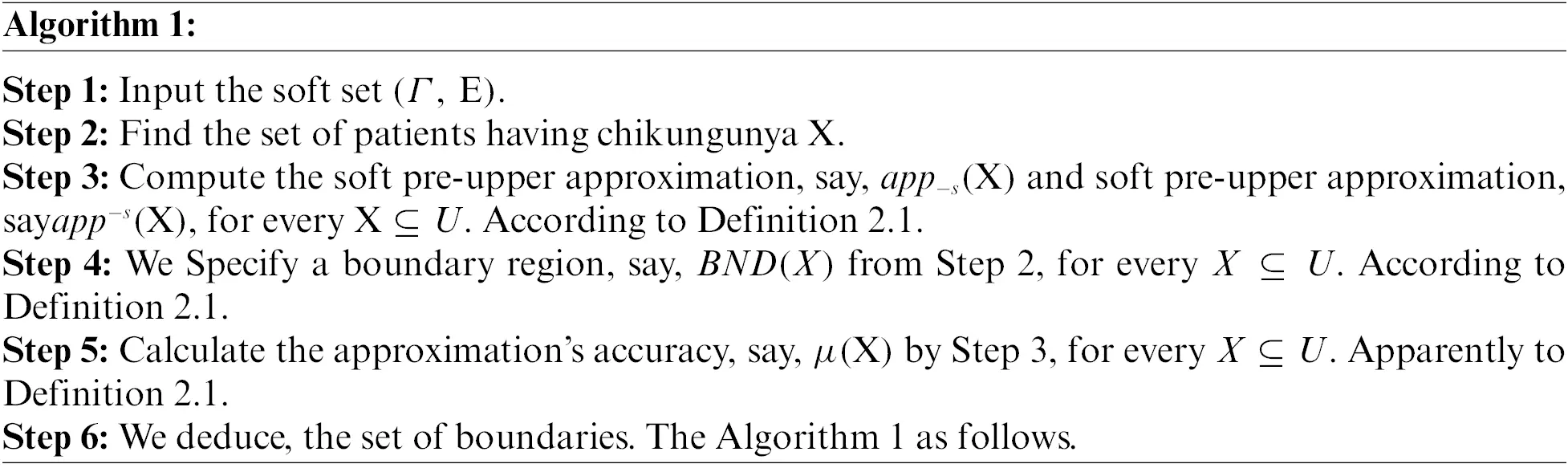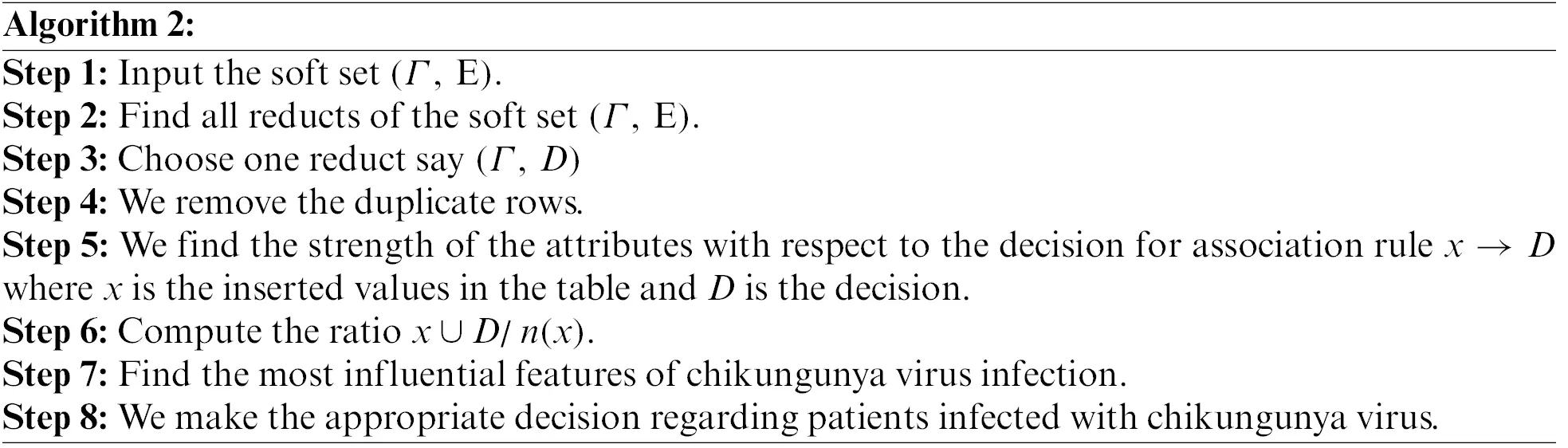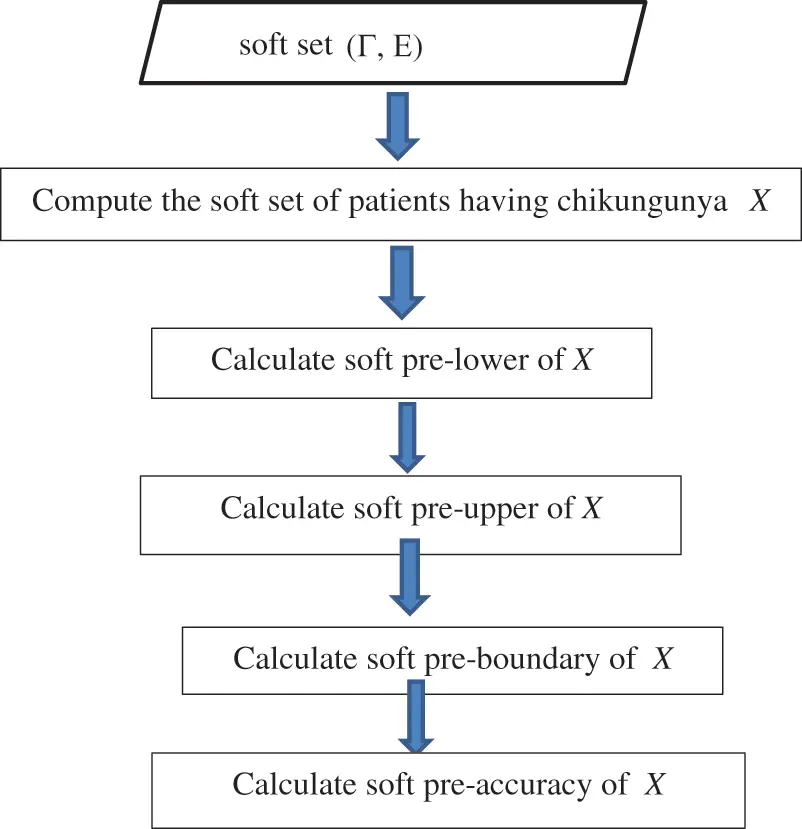Figure 1: Framework of the proposed method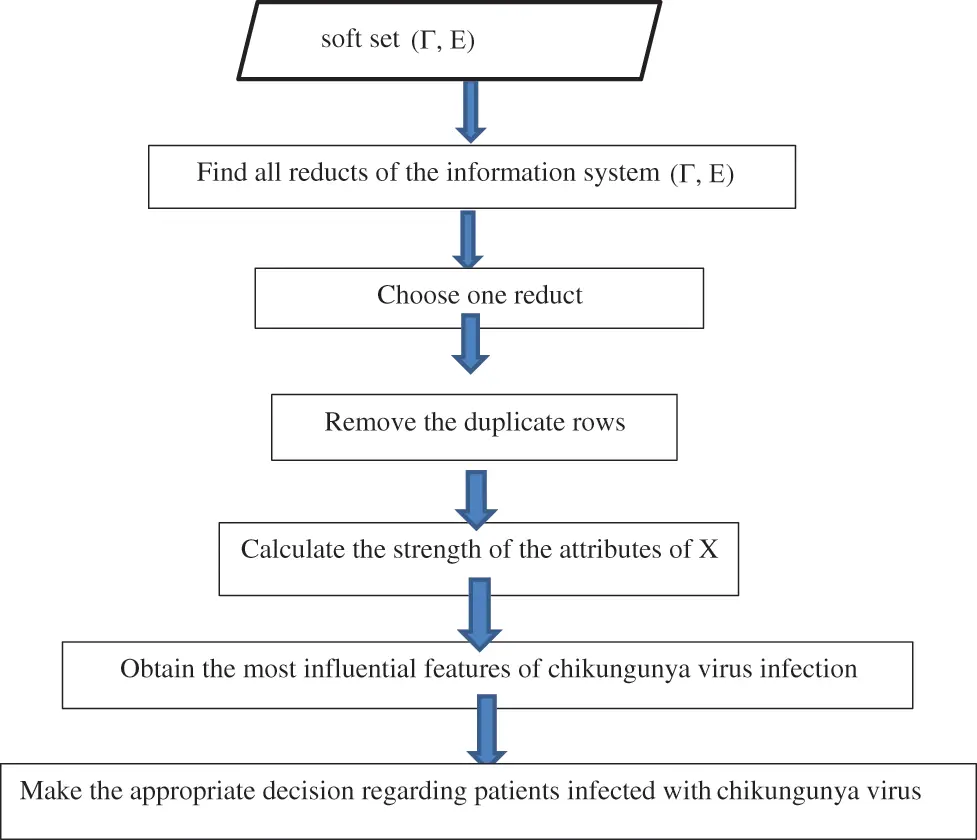Figure 2: Framework of the result of the proposed method

4  Chikungunya Medical Application

Here we explore the problem of chikungunya, a disease that has been spread by Aedes mosquitoes that carry a virus that infects humans. CHIKV epidemics have occurred recently, linked to serious diseases. It generates a high temperature as well as significant joint discomfort. Muscle discomfort, headaches, and nausea are some of the other symptoms. The first signs and symptoms are similar to those of dengue fever. It typically does not endanger one's life. However, joint discomfort might linger for a long time. It might take months for you to fully heal. In most cases, the patient develops lifetime immunity to infection, making re-infection extremely unlikely. The illness has expanded throughout Africa and Asia in recent decades, particularly the subcontinent of India. Observe the table below, which contains information on 8-patients.

The following is a description of a set-valued information system in Table 1: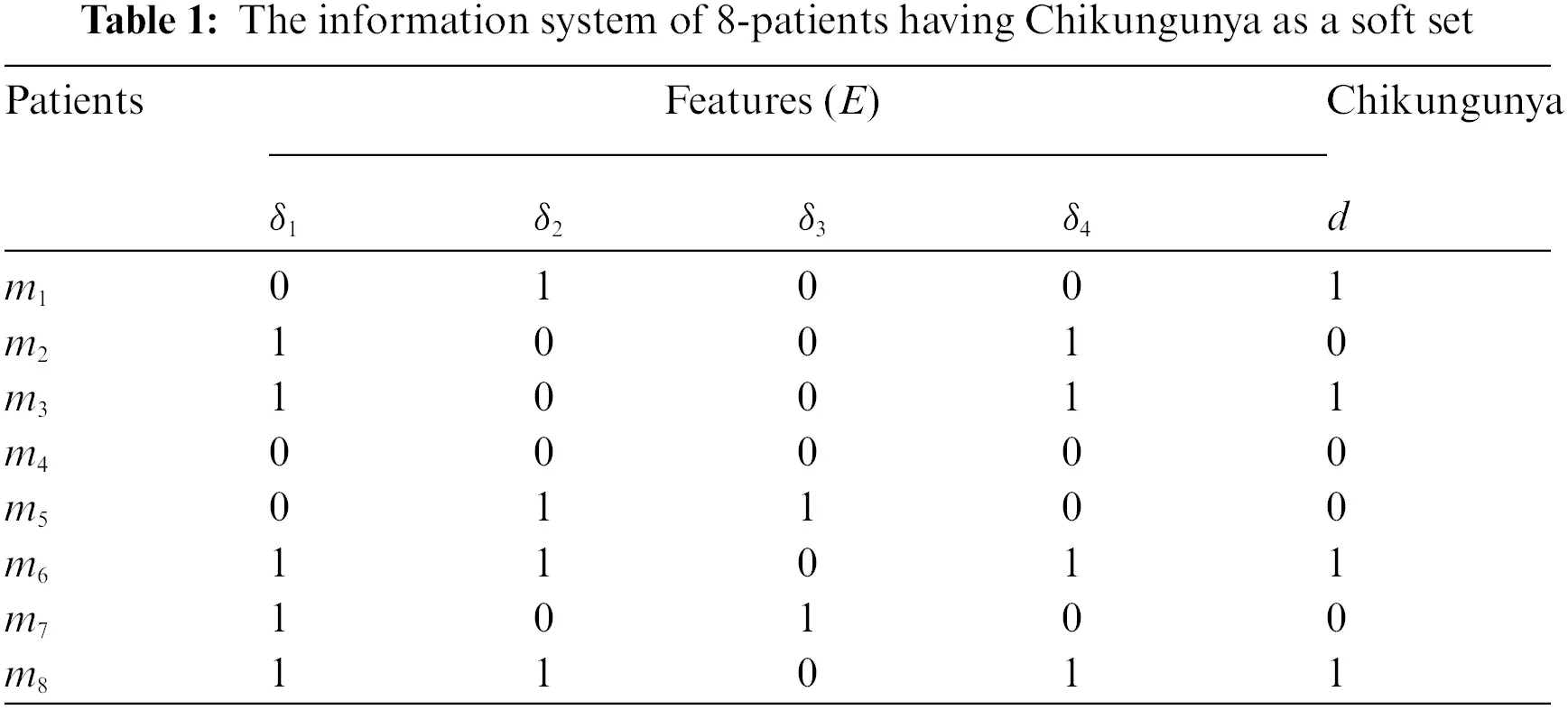H={m1,m2,m3,m4,m5,m6,m7,m8} of 8-patients, E={δ_1 = Nausea, δ_2 = Headache, δ3 = Joint pain, δ4 = Temperature} be a set of parameters which illustrate symptoms for patients. Consider the soft set (Γ,E), where (Γ,E)={(δ1,{m2,m3,m6,m7,m8}),(δ2,{m1,m5,m6,m8}),(δ3,{m5,m7}),(δ4,{m2,m3,m6,m8})}which describes having chikungunya and having not chikungunya as in the following Table 1.

Let Y={m2,m4,m5,m7} is the set of patients having not Chikungunya, the lower and the upper of this set are by our method is BS(Y)={m2,m5,m7},Bs(Y)=H and the boundary BND(Y)={m1,m3,m4,m6,m8} and the accuracy is μA(Y)=3/8 since the patients {m2,m4,m5,m7} having not Chikungunya or {m1,m3,m6,m8} are the patients having Chikungunya, since the boundary region {m1,m3,m4,m6,m8}, hence the patients m1,m3,m4,m6 and m8 cannot be categorized in a unique way in view of the current state of knowledge. The patients m1,m5 and m7 have indications that allow us to categorize them as chikungunya patients with precision. We can consider the indications which enable us to categorize them with confidence as Chikungunya, joint pain, Nausea, headache and temperature as the conditions we consider Chikungunya because not all condition features in a data system are required to describe features before generating judgment rules. It is possible that the judgment features rely on only a subset of condition features. Thus, we are interested in identifying this subset, which is provided by the core where H={m1,m2,m3,m4,m5,m6,m7,m8}. Then, the reduct of these problems are {δ1,δ2,δ4} and {δ1,δ3,δ4}.

5  Rule Generation

In this section, the rules will be generated depending on the reduct. and core as in Table 1, since the reduct sets are {δ1,δ3,δ4} and {δ2,δ3,δ4} also, the core sets are δ3, and δ4. We can find the strength of the attributes with respect to the decision for an association rule xD which is the ration of number of the problems that contains x . From Table 1, we calculate the strength of the attributes {δ1,δ2,δ4} as follows:

(δ1=0)D=1 the strength of this particular rule is revealed to be 33%

(δ1=1)D=0 the strength of this particular rule is revealed to be 20%

(δ1=1)D=1 the strength of this particular rule is revealed to be 60%

(δ1=0)D=0 the strength of this particular rule is revealed to be 67%

Similarly, the strength of rule δ2 can be found as follows: (δ2=1)D=1 the strength of this particular rule is revealed to be 75%.

(δ2=0)D=0 the strength of this particular rule is revealed to be 75%

(δ2=0)D=1 the strength of this particular rule is revealed to be 25%

(δ2=1)D=0 the strength of this particular rule is revealed to be 25%

Similarly, the strength of rule δ3 can be found as follows:

(δ3=0)D=1 the strength of this particular rule is revealed to be 67%

(δ3=0)D=0 the strength of this particular rule is revealed to be 33%

(δ3=1)D=0 the strength of this particular rule is revealed to be 100%

Finally, we can find the strength of rules δ4 as follows:

(δ4=0)D=1 the strength of this particular rule is revealed to be 25%

(δ4=1)D=0 the strength of this particular rule is revealed to be 25%

(δ4=1)D=1 the strength of this particular rule is revealed to be 75%

(δ4=0)D=0 the strength of this particular rule is revealed to be 100%

Then, from the above calculations we find that the attributes δ3 and δ4 are more affected than by Chikungunya from the other attributes because the strength of the rules for the attributes are maximum then from Table 1, the reduct sets are {δ2,δ3,δ4}, {δ1,δ3,δ4} and the core is {δ3,δ4} we can be reduced to the Table 2. We note that, IND (E) ≠ IND (E – {δ3}), …., then δ3, and δ4 are indispensable. Also, we get δ1 removed then we obtain IND (E) = IND (E– {δ1}), and superfluous are δ1, δ2.

Core attributes one removal with MATLAB program . Next, we get Remove Themes as the next Table 2.

From this Table 2, we find the core of this table in such a method remains consistent if we delete the value δ4=0. We find there are two decision values 1 and 0; this means that the based-on attribute δ4 and we cannot make a unique decision. Thus, the values cannot be deleted. Similarly, if we delete δ3=0 we find there are two decision values 1 and 0. Based on attribute δ3, we cannot make a unique decision; thus, the values cannot be deleted, as in the following Table 3.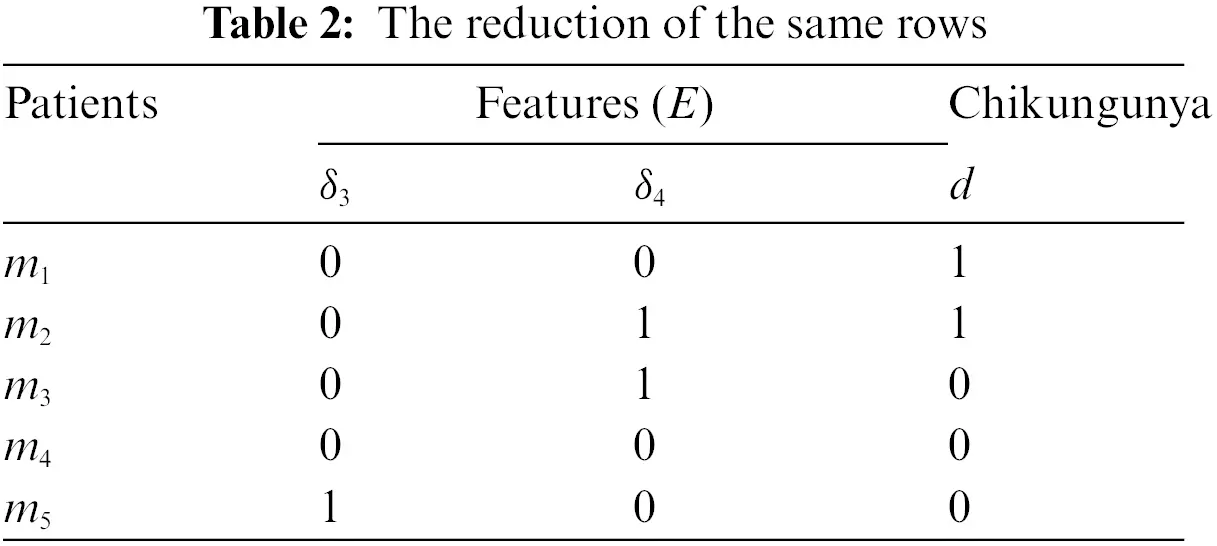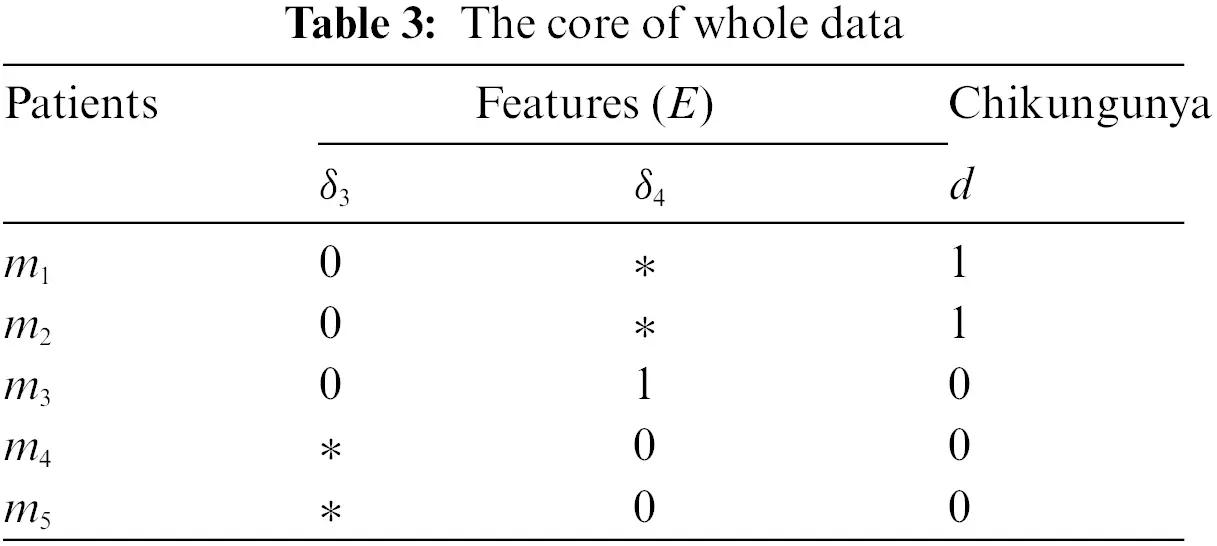Thus, Table 3 shows that the core of the whole Table 1 This enables us to reduce to Table 3 by merging duplicate rows. We can eliminate the identical rows and get the following Table 4.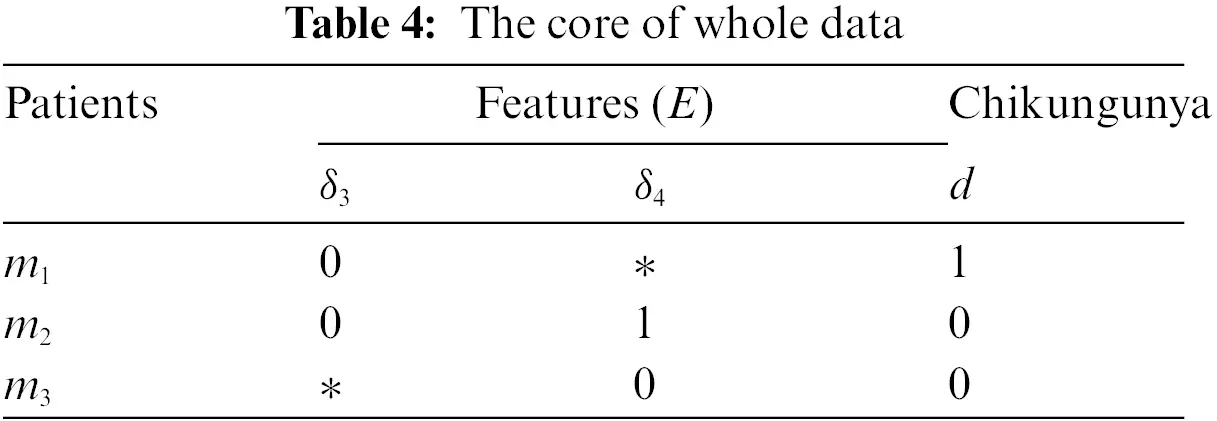Table 4 gives us the decision rules based on the reduction as follows: if (δ1=0) then D=1(δ3=0) and δ4=1 then D=0 and if (δ4=0) then D=0. From our approach, determining the most important attribute based on the strength of this application can be used in many fields of knowledge discovery data mining.

6  Conclusion

In this article, we introduce the characteristics of the approach soft pre-rough set approximation and its decision making. We have introduced a new definition of this approach namely, soft pre-rough equality, soft pre-rough inclusion, soft pre-lower, soft pre-upper belong, soft pre-dense, soft pre-nowhere dense, soft pre-residual, soft pre-external lower and soft pre-internal upper and we also study some of their properties. We used our novel approach to identify the most important trait on the basis of its strength, which is an important method for analytical approach and decision making for any real-life problems. Also, we made a medical application to illustrate our method. This application can be used on any number of patients, any life problem and comment on the decision. Finally, the applicable technique is applied to a case study of a topological concept development strategy from the perspective of chikungunya virus in nature to validate the proposed method, as well as some comparison evaluations. We have explained our method with two algorithms and how to apply it using MATLAB. In reality, our suggestion is helpful in solving any future real-life problems. In the future, we shall extend the proposed methods to a variety of other concepts, such as the fuzzy set and fuzzy rough set.

Acknowledgement: The authors are thankful to the Deanship of Scientific Research at Najran University for funding this work under the General Research Funding Program Grant Code (NU/-/SERC/10/603).

Funding Statement: This research work is supported by the Deanship of the Scientific Research at Najran University, Najran, Saudi Arabia [NU/-/SERC/10/603].

Conflicts of Interest: The authors declare that they have no conflicts of interest to report regarding the present study.

## References

1. El Safty, M. A. (2021). Modeling uncertainty knowledge of the topological methods. Poincare Journal of Analysis & Applications, 8(1), 1-14. [Google Scholar] [CrossRef]
2. El Safty, M. A., El Sayed, M., & Alblowi, S. A. (2021). Accuracy based on simply* alpha open set in rough set and topological space. Soft Computing, 25(16), 10609-10615. [Google Scholar] [CrossRef]
3. Ali, A., Ali, M. I., & Rehman, N. (2019). Soft dominance based rough sets with applications in information systems. International Journal of Approximate Reasoning, 113, 171-195. [Google Scholar] [CrossRef]
4. Molodtsov, D. A. (1999). Soft set theory-first results. Computers & Mathematics with Applications, 37(4–5), 19-31. [Google Scholar] [CrossRef]
5. Babitha, K. V., Sunil, J. J. (2016). Soft topologies generated by soft set relations. In: Handbook of research on generalized and hybrid set structures and applications for soft computing, pp. 118–126. Calicut, India: Academic Press. DOI 10.4018/978-1-4666-9798-0.ch007. [CrossRef]
6. El-Sayed, M., & El-Bably, M. K. (2017). Soft simply open set in soft topological space. Journal of Computational and Theoretical Nanoscience, 14(8), 4104-4113. [Google Scholar] [CrossRef]
7. El Sayed, M. A., Alblowi, S. A., Almalki, Y., & El Sayed, M. (2022). Coronavirus decision-making based on a locally simply*alpha generalized closed set. Intelligent Automation & Soft Computing, 32(1), 483-498. [Google Scholar] [CrossRef]
8. El Sayed, M., Al Qubati, A. Q., & El-Bably, M. K. (2020). Soft pre-rough sets and its applications in decision making. Mathematical Biosciences and Engineering, 17(5), 6045-6063. [Google Scholar] [CrossRef]
9. Shabir, M., Ali, M. I., & Shaheen, T. (2013). Another approach to soft rough sets. Knowledge-Basis Systems, 40, 72-80. [Google Scholar] [CrossRef]
10. El Safty, M. A., AL Zahrani, S., El-Bably, M. K., & El Sayed, M. (2022). Soft xi-rough set and its applications in decision making of coronavirus. Computers, Materials & Continua, 70(1), 267-285. [Google Scholar] [CrossRef]
11. Sai, B. V. S. T. (2020). Applications of soft set-in decision-making problems. Global Journal of Pure and Applied Mathematics, 16(2), 305-324. [Google Scholar] [CrossRef]
12. Ping, J., Atef, N., Khalil, A. M., Riaz, M., & Hassan, N. (2021). Soft rough q-rung orthopair m-polar fuzzy sets and q-rung orthopair m-polar fuzzy soft rough sets and their applications. IEEE Access, 9, 139186-139200. [Google Scholar] [CrossRef]
13. Sahu, R., Dash, S. R., & Das, S. (2021). Career selection of students using hybridized distance measure based on picture fuzzy set and rough set theory. Decision Making: Applications in Management and Engineering, 4(1), 104-126. [Google Scholar] [CrossRef]
14. Liu, Y., Qin, K., & Martínez, L. (2018). Improving decision making approaches based on fuzzy soft sets and rough soft sets. Applied Soft Computing, 65, 320-332. [Google Scholar] [CrossRef]
15. Maji, P. K., Biswas, R., & Roy, A. R. (2003). Soft set theory. Computers and Mathematics with Applications, 45(4–5), 555-566. [Google Scholar] [CrossRef]
16. Maji, P. K., Roy, A. R., & Biswas, R. (2002). An application of soft sets in a decision-making problem. Computer Mathematical Applications, 44, 1077-1083. [Google Scholar] [CrossRef]
17. Feng, F., Liu, X., Fotea, V. L., & Jun, Y. B. (2011). Soft sets and soft rough sets. Information Sciences, 181(6), 1125-1137. [Google Scholar] [CrossRef]
18. Pawlak, Z. (1982). Rough sets. International Journal of Information Computer Science, 11(5), 341-356. [Google Scholar] [CrossRef]
19. Ali, M. I. (2011). A note on soft sets, rough soft sets and fuzzy soft sets. Applied Soft Computing, 11, 3329-3332. [Google Scholar] [CrossRef]
20. Sharma, H. K., Kumari, K., & Kar, S. (2020). A rough set approach for forecasting models. Decision Making Applications in Management and Engineering, 3(1), 1-21. [Google Scholar] [CrossRef]
21. Sun, Q. M., Zhang, Z. L., & Liu, J. (2008). Soft sets and soft modules. Rough Sets and Knowledge Technology, 5009, 403-409. [Google Scholar] [CrossRef]
22. El Safty, M. A., Mousa, A. A., Alblowi, S. A., & El Sayed, M. (2020). Topological approach on fuzzy soft. Nanoscience and Nanotechnology Letters, 12(11), 1323-1328. [Google Scholar] [CrossRef]
23. Tanay, B., & Kandemir, M. B. (2011). Topological structure of fuzzy soft sets. Computers and Mathematics with Application, 61(10), 2952-2957. [Google Scholar] [CrossRef]
24. Shakiba, A., Hooshmandasl, M. R., Davvaz, B., & Fazeli, S. A. (2017). S-Approximation spaces: A fuzzy approach. Iranian Journal of Fuzzy Systems, 14(2), 127-154. [Google Scholar] [CrossRef]
25. Alblowi, S. A., El Sayed, M., & El Safty, M. A. (2021). Decision making based on fuzzy soft sets and its application in COVID-19. Intelligent Automation & Soft Computing, 30(3), 961-972. [Google Scholar] [CrossRef]
26. El Safty, M. A., AL Zahrani, S., Ahmar, A. S., & El Sayed, M. (2022). Decision making on fuzzy soft simply* continuous of fuzzy soft multi-function. Computer Systems Science and Engineering, 40(3), 881-894. [Google Scholar] [CrossRef]This work is licensed under a Creative Commons Attribution 4.0 International License, which permits unrestricted use, distribution, and reproduction in any medium, provided the original work is properly cited.